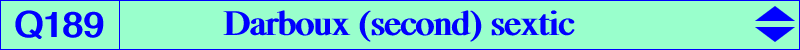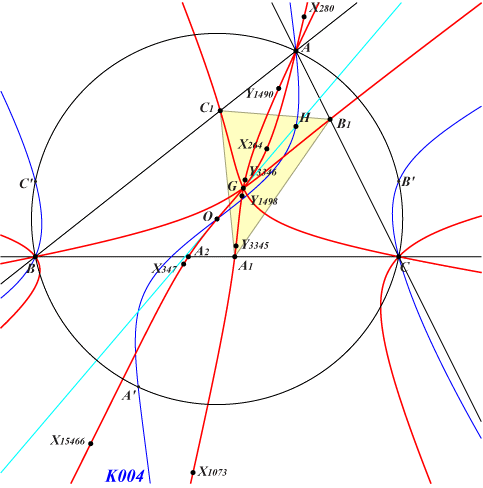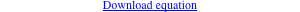too complicated to be written here. Click on the link to download a text file.X(2), X(3), X(264), X(280), X(347), X(1073), X(15466) A1, B1, C1 : vertices of the cevian triangle of X(264) A2, B2, C2 : vertices of the cevian triangle of X(15466) extraversions of X(280), X(347) other points belowQ172 answers a question raised by Sriram Panchapakesan and Antreas Hatzipolakis rephrased as follows. Let ABC be a triangle, P a point and PaPbPc the circumcevian triangle of P. Let X = BC ∩ OPa, Y = CA ∩ OPb, Z = AB ∩ OPc. ABC and XYZ are perspective (at Q) if and only if P lies on the Darboux cubic K004. The locus of the perspector Q, as P moves on the Darboux cubic, is the Darboux sextic Q172. When P1, P2 are two isogonal conjugate points on K004 (hence collinear with X20), then the perspectors Q1, Q2 are two isotomic conjugate points, hence Q172 is a self-isotomic curve. When P1, P2 are two points on K004, symmetric about O, then Q1, Q2 and G are collinear. *** G is a quadruple point on Q172 and the tangents pass through X(92) and its extraversions. A, B, C are nodes and the tangents at these points are the cevian lines of X(3) and X(1073). Note that the tangents at O, A1, B1, C1 pass through G. Q172 is a strong curve hence it contains the extraversions of every weak point on the curve. These are the perspectors of the weak points on K004. *** Other points on Q172 (when P = Xi on K004, the corresponding perspector is denoted by Yi). Y1490 = (a-b-c) (a^3-a^2 b-a b^2+b^3+a^2 c+2 a b c+b^2 c-a c^2-b c^2-c^3) (a^3+a^2 b-a b^2-b^3-a^2 c+2 a b c-b^2 c-a c^2+b c^2+c^3) (a^6-2 a^5 b-a^4 b^2+4 a^3 b^3-a^2 b^4-2 a b^5+b^6-2 a^5 c-2 a^4 b c+2 a b^4 c+2 b^5 c-a^4 c^2+2 a^2 b^2 c^2-b^4 c^2+4 a^3 c^3-4 b^3 c^3-a^2 c^4+2 a b c^4-b^2 c^4-2 a c^5+2 b c^5+c^6) : : , SEARCH = 3.91324419095623. Y1498 = a^2 (a^2-b^2-c^2) (a^4-2 a^2 b^2+b^4+2 a^2 c^2+2 b^2 c^2-3 c^4) (a^4+2 a^2 b^2-3 b^4-2 a^2 c^2+2 b^2 c^2+c^4) (a^8-4 a^6 b^2+6 a^4 b^4-4 a^2 b^6+b^8-4 a^6 c^2-4 a^4 b^2 c^2+4 a^2 b^4 c^2+4 b^6 c^2+6 a^4 c^4+4 a^2 b^2 c^4-10 b^4 c^4-4 a^2 c^6+4 b^2 c^6+c^8) : : , SEARCH = 5.91013578043065. Y3345 = (a+b-c) (a-b+c) (a^3+a^2 b-a b^2-b^3+a^2 c-2 a b c+b^2 c-a c^2+b c^2-c^3) (a^6+2 a^5 b-a^4 b^2-4 a^3 b^3-a^2 b^4+2 a b^5+b^6-2 a^5 c+2 a^4 b c+2 a b^4 c-2 b^5 c-a^4 c^2+2 a^2 b^2 c^2-b^4 c^2+4 a^3 c^3+4 b^3 c^3-a^2 c^4-2 a b c^4-b^2 c^4-2 a c^5-2 b c^5+c^6) (a^6-2 a^5 b-a^4 b^2+4 a^3 b^3-a^2 b^4-2 a b^5+b^6+2 a^5 c+2 a^4 b c-2 a b^4 c-2 b^5 c-a^4 c^2+2 a^2 b^2 c^2-b^4 c^2-4 a^3 c^3+4 b^3 c^3-a^2 c^4+2 a b c^4-b^2 c^4+2 a c^5-2 b c^5+c^6) : : , SEARCH = 1.08396830717217 Y3346 = b^2 c^2 (-a^2+b^2-c^2) (a^2+b^2-c^2) (-3 a^4+2 a^2 b^2+b^4+2 a^2 c^2-2 b^2 c^2+c^4) (a^8+4 a^6 b^2-10 a^4 b^4+4 a^2 b^6+b^8-4 a^6 c^2+4 a^4 b^2 c^2+4 a^2 b^4 c^2-4 b^6 c^2+6 a^4 c^4-4 a^2 b^2 c^4+6 b^4 c^4-4 a^2 c^6-4 b^2 c^6+c^8) (a^8-4 a^6 b^2+6 a^4 b^4-4 a^2 b^6+b^8+4 a^6 c^2+4 a^4 b^2 c^2-4 a^2 b^4 c^2-4 b^6 c^2-10 a^4 c^4+4 a^2 b^2 c^4+6 b^4 c^4+4 a^2 c^6-4 b^2 c^6+c^8) : : , SEARCH = -39.9574617338905. These points are now X(46350), X(46351), X(46352), X(46353) in ETC (2021-12-13). *** A property by Elias M. Hagos (see Euclid #3515) For P on the Darboux cubic K004, the antipedal triangle of the isogonal conjugate of P and ABC are perspective at T on the Thomson cubic K002 (see K004, property 1). Then Q is the barycentric quotient P ÷ T. If P = x:y:z, then Q = b^2 c^2 x (SB SC x^2 + a^2 SA (y^2 + z^2) + 2a^2 SO y z - S^2 x (y + z) + 2 a^2 x (c^2 y + b^2 z)) : : .P 1 3 4 20 40 64 84 1490 1498 3182 3183 3345 3346 3347 3353 T 1 2 6 3 9 4 57 223 1249 3341 3343 282 1073 3342 3351 Q 2 3 264 15466 347 1073 280 Y1490 Y1498 Y3345 Y3346*** Generalization K004 is a special case of circumcevian pK as studied in CL072, see specially PK(-1). Every such cubic, denoted by K(S), is obtained when O above is replaced by a point S ≠ X6. With S = X1, X2, X4, X21 we find the listed cubics K318, K959, K919, K1173 respectively. With S = X6, the cubic decomposes into the union of the symmedians, excluded in the sequel. Each cubic is associated with a sextic ∑(S), analogous to Q172 = ∑(O), with a quadruple point at the cevapoint S0 of X(6) and S. This sextic is invariant under the isoconjugation (denoted by *) with fixed point S0 hence pole S0^2. K(S) = pK(X6 x S0, agS x S0), where "x" denotes a barycentric product and agS is the anticomplement of isogonal conjugate of S. ∑(S) passes through the following points : • S1 = S ÷ agS (barycentric quotient of S and agS), • S2 = S*, • S3 = S1*, • S4 = S x a[(gS)^2] ÷ S, • S5 = S4*, • vertices of the cevian triangles of S2 and S3, • A, B, C which are double points with nodal tangents passing through S0 and S1. With S = u : v : w, the equation of ∑(S) is given here. See Q173 = ∑(X1) and Q174 = ∑(X55), an isogonal sextic.